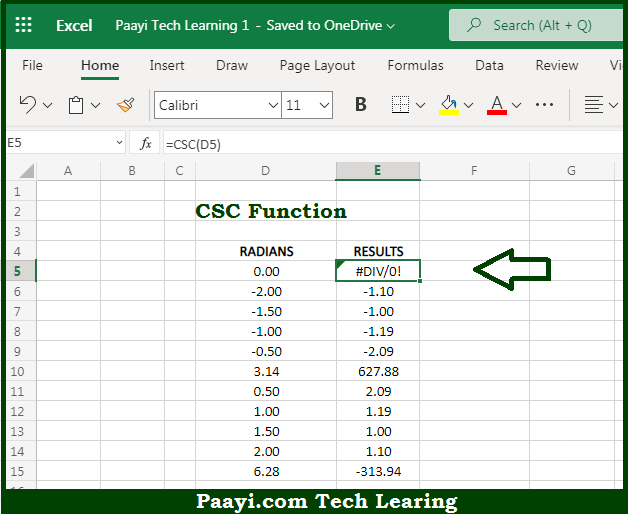# Learn How to Use Microsoft Excel CSC Function

Written by | 0 Comments | 602 Views

In this article, you will learn how to use the Microsoft Excel CSC function and its prime function in Microsoft Excel. You will also get to know the Microsoft Excel CSC function return value and syntax with the help of some examples.

Microsoft Excel CSC Function

The main purpose of the Microsoft Excel CSC function is to get the cosecant of the angle. That implies, with the help of the CSC function you can able to return the cosecant of the angle provided in radians. In geometry, the cosecant of an angle is equal to the ratio of a right triangle's hypotenuse divided by its opposite side. So, with the help of the CSC function, you can able to get the cosecant of the provided angle.

Return Value of CSC Function

The return value will be the cosecant value.

Syntax of CSC Function

=CSC(number)

Where the arguments:

• number: This is the angle provided in radians.

## How to Use Microsoft Excel CSC Function?So we know that Microsoft Excel CSC function you can able to get the cosecant of the angle. That implies, with the help of the CSC function you can able to return the cosecant of the angle provided in radians. In geometry, the cosecant of an angle is equal to the ratio of a right triangle's hypotenuse divided by its opposite side. So, with the help of the CSC function, you can able to get the cosecant of the provided angle.